# RD Sharma Solutions for Class 10 Maths Chapter 4 Triangles Exercise 4.4

More on the basic proportionality theorem is discussed in this exercise. Few problems to strengthen the key concept are done by solving in the right manner. To know how to present and make your solutions mistake-free, students can access the RD Sharma Solutions Class 10. Students aspiring to score high marks can download the RD Sharma Solutions for Class 10 Maths Chapter 4 Triangles Exercise 4.4 PDF provided below.

## RD Sharma Solutions for Class 10 Maths Chapter 4 Triangles Exercise 4.4 Download PDF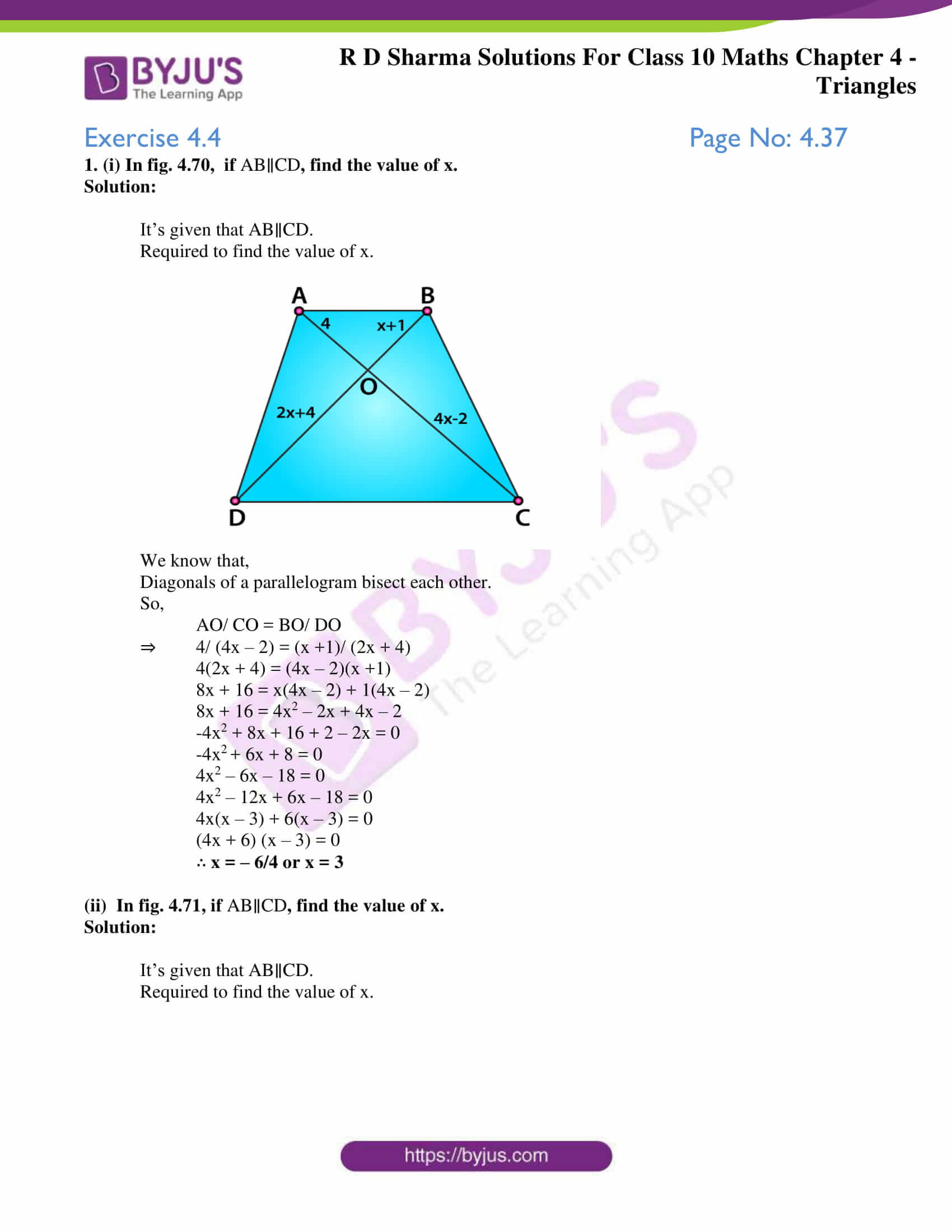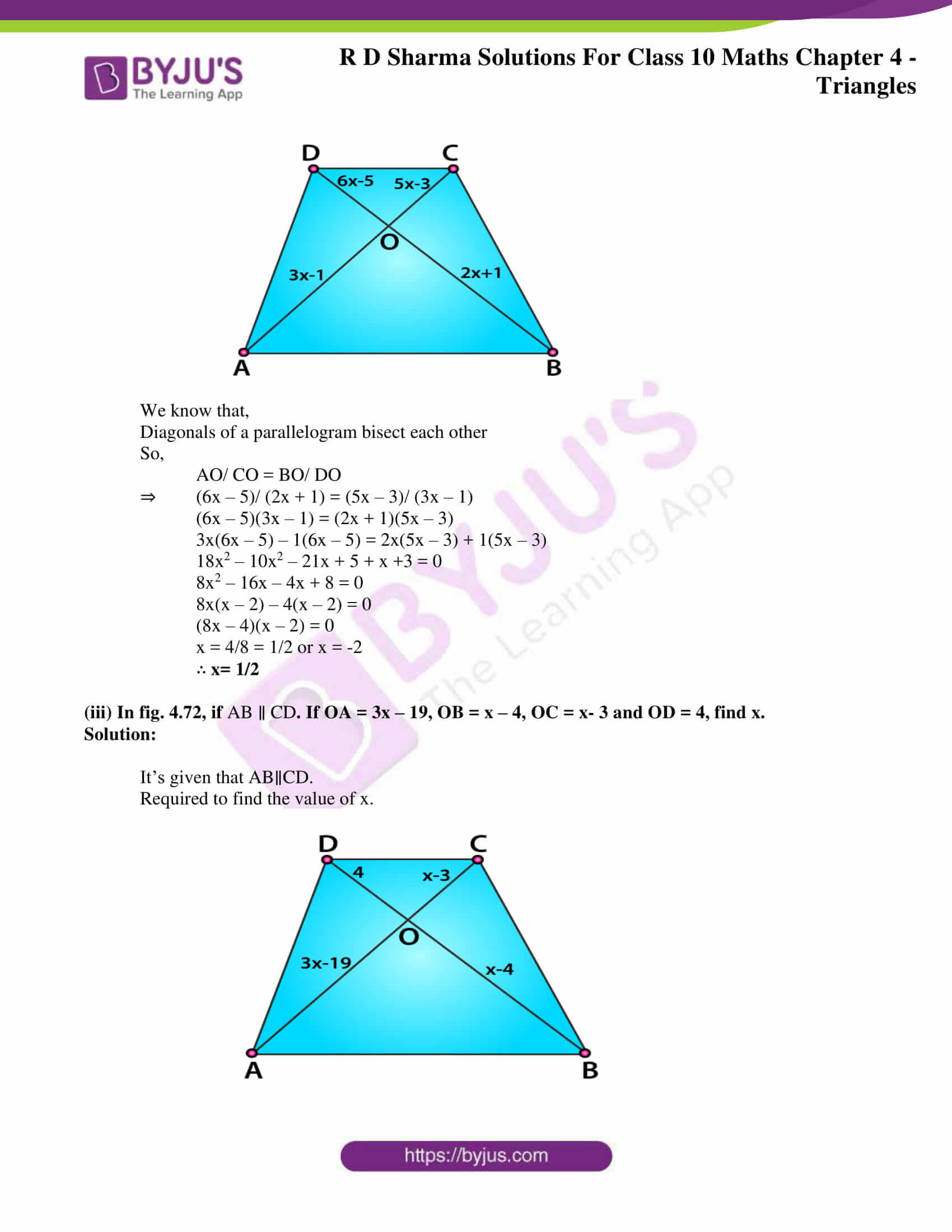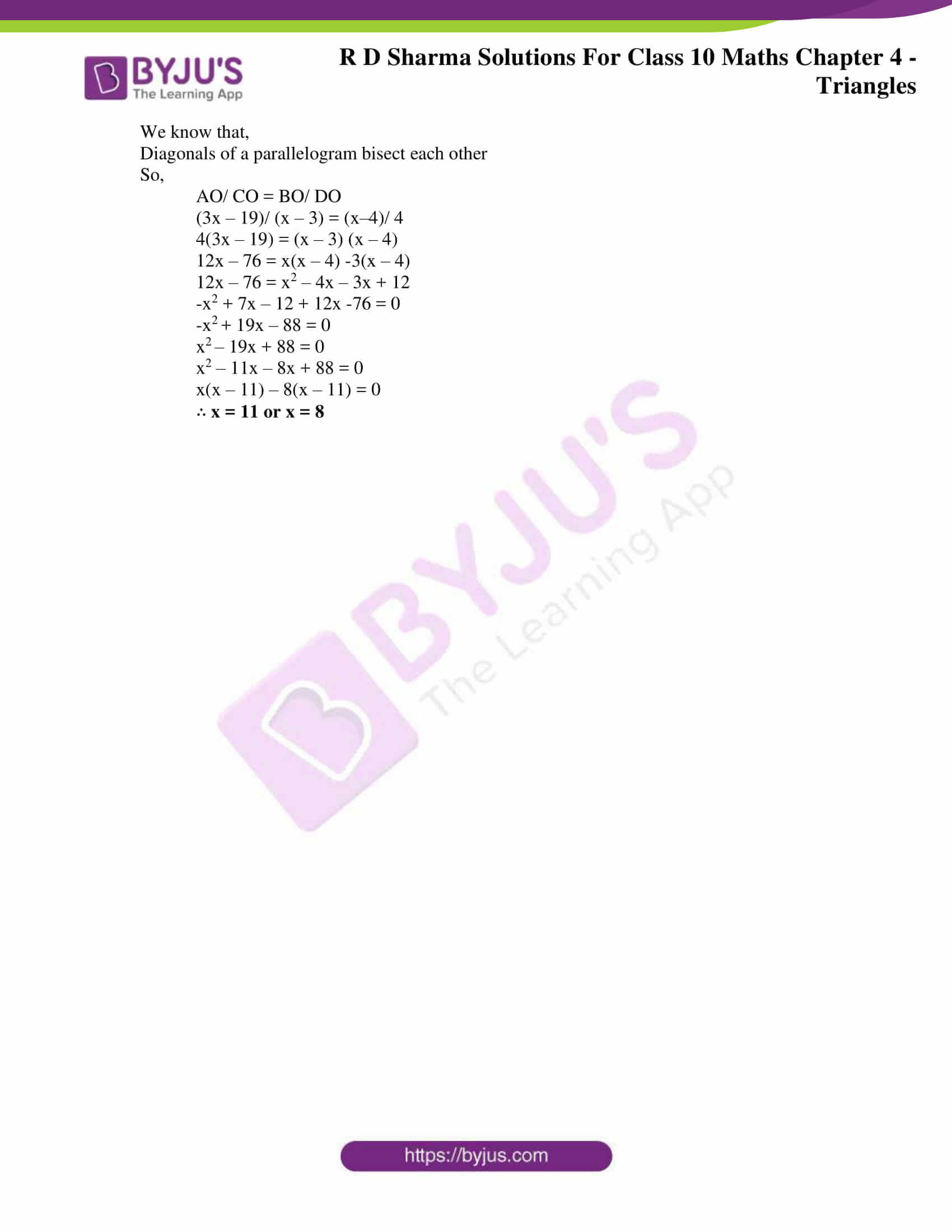### Access RD Sharma Solutions for Class 10 Maths Chapter 4 Triangles Exercise 4.4

1. (i) In fig. 4.70, if AB∥CD, find the value of x.

Solution:

It’s given that AB∥CD.

Required to find the value of x.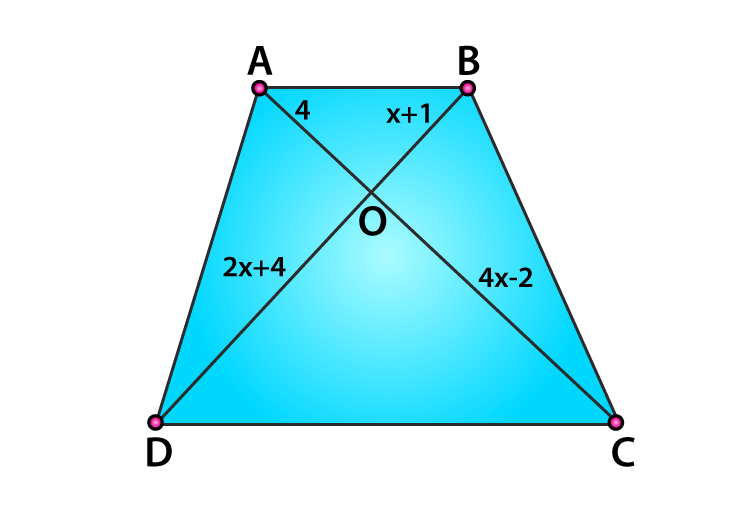We know that,

Diagonals of a parallelogram bisect each other.

So,

AO/ CO = BO/ DO

⇒ 4/ (4x – 2) = (x +1)/ (2x + 4)

4(2x + 4) = (4x – 2)(x +1)

8x + 16 = x(4x – 2) + 1(4x – 2)

8x + 16 = 4x2 – 2x + 4x – 2

-4x2 + 8x + 16 + 2 – 2x = 0

-4x+ 6x + 8 = 0

4x2 – 6x – 18 = 0

4x2 – 12x + 6x – 18 = 0

4x(x – 3) + 6(x – 3) = 0

(4x + 6) (x – 3) = 0

x = – 6/4 or x = 3

(ii)  In fig. 4.71, if AB∥CD, find the value of x.

Solution:

It’s given that AB∥CD.

Required to find the value of x.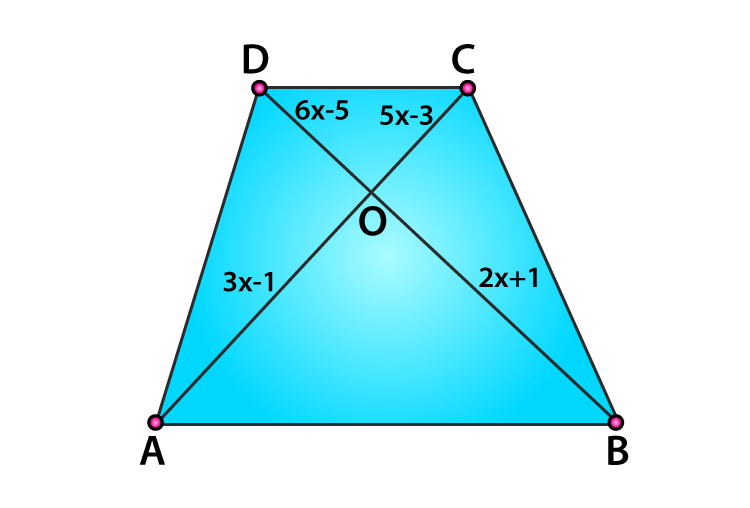We know that,

Diagonals of a parallelogram bisect each other

So,

AO/ CO = BO/ DO

⇒ (6x – 5)/ (2x + 1) = (5x – 3)/ (3x – 1)

(6x – 5)(3x – 1) = (2x + 1)(5x – 3)

3x(6x – 5) – 1(6x – 5) = 2x(5x – 3) + 1(5x – 3)

18x2 – 10x2 – 21x + 5 + x +3 = 0

8x2 – 16x – 4x + 8 = 0

8x(x – 2) – 4(x – 2) = 0

(8x – 4)(x – 2) = 0

x = 4/8 = 1/2 or x = -2

x= 1/2

(iii) In fig. 4.72, if AB ∥ CD. If OA = 3x – 19, OB = x – 4, OC = x- 3 and OD = 4, find x.

Solution:

It’s given that AB∥CD.

Required to find the value of x.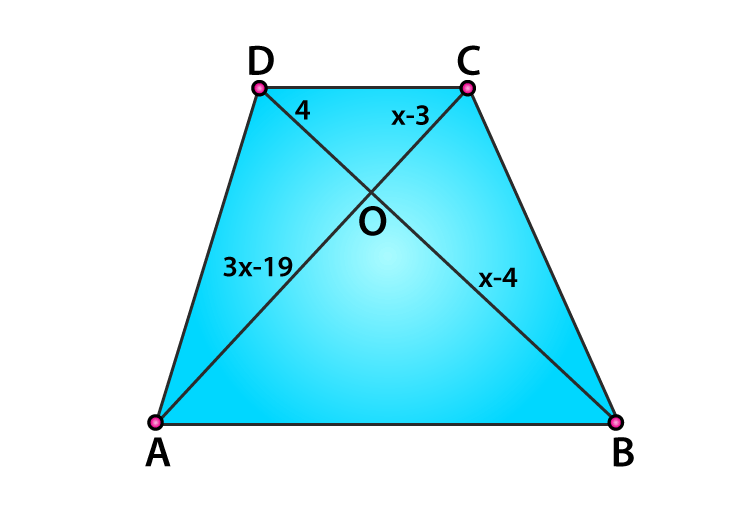We know that,

Diagonals of a parallelogram bisect each other

So,

AO/ CO = BO/ DO

(3x – 19)/ (x – 3) = (x–4)/ 4

4(3x – 19) = (x – 3) (x – 4)

12x – 76 = x(x – 4) -3(x – 4)

12x – 76 = x2 – 4x – 3x + 12

-x2 + 7x – 12 + 12x -76 = 0

-x+ 19x – 88 = 0

x– 19x + 88 = 0

x2 – 11x – 8x + 88 = 0

x(x – 11) – 8(x – 11) = 0

x = 11 or x = 8